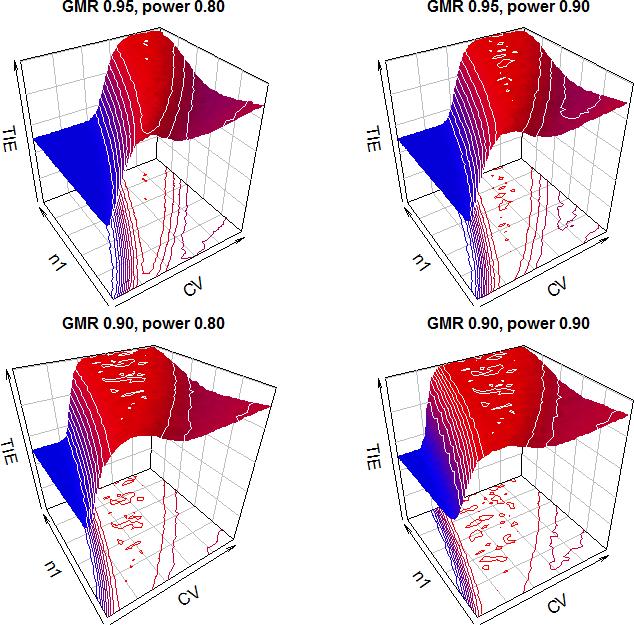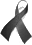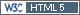## Finally: Exact TSD methods for 2×2 crossover designs [Two-Stage / GS Designs]

Dear all,

in version 0.5-1 of package `Power2Stage` exact methods1,2,3 are implemented – after months of struggling (many THX to Ben). The methods are extremely flexible (arbitrary BE-limits and target power, futility criteria on the PE, its CI, and the maximum total sample size, adapting for the PE of stage 1).

I’ve heard in the past that regulatory statisticians in the EU prefer methods which strictly control the Type I Error (however, at the 3rd GBHI conference in Amsterdam last week it was clear that methods based on simulations are perfectly fine for the FDA) and the inverse normal method with repeated confidence intervals would be the method of choice. Well roared lion; wasn’t aware of software which can to this job. That’s like saying “Fly to Mars but you are not allowed to use a rocket!” What else? Levitation? Witchcraft? Obtaining two p-values (like in TOST) is fairly easy but to convert them into a confidence interval (as required in all guidelines) not trivial.
Despite we showed this approach4 a while ago, nothing was published in a peer-reviewed journal until very recently.
Although we have a method now which demonstrated to control the TIE, I was curious how it performs in simulations (just to set it into perspective).
R-code at the end of the post (with small step sizes of CV and n1 expect runtimes of some hours; in large simulations I don’t recommend `pmethod="exact"` – about 10times slower than `pmethod="nct"`). See the documentation of function `power.tsd.in()` about how to set futility criteria and make it fully adaptive. As usual in the latter case say goodbye to power…

I explored scenarios, maximum combination test
1. Conventional BE-limits, GMR 0.95, target power 0.90, CV 0.10–0.60, n1 12–72, no futility
```print(x\$TIE.max, row.names=FALSE)    CV n1 alpha maximum TIE signif  0.21 56  0.05     0.05025     no print(x\$power.min, row.names=FALSE)   CV n1 target minimum power  0.6 12    0.8        0.7902```
2. Conventional BE-limits, GMR 0.95, target power 0.80, CV 0.10–0.60, n1 12–72, no futility
```print(x\$TIE.max, row.names=FALSE)    CV n1 alpha maximum TIE signif  0.38 48  0.05    0.050164     no print(x\$power.min, row.names=FALSE)   CV n1 target minimum power  0.6 12    0.9       0.86941```
3. Conventional BE-limits, GMR 0.90, target power 0.80, CV 0.10–0.60, n1 12–72, no futility
```print(x\$TIE.max, row.names=FALSE)    CV n1 alpha maximum TIE signif  0.32 44  0.05      0.0503     no print(x\$power.min, row.names=FALSE)   CV n1 target minimum power  0.6 12    0.9      0.78578```
4. Conventional BE-limits, GMR 0.90, target power 0.90, CV 0.10–0.60, n1 12–72, no futility
```print(x\$TIE.max, row.names=FALSE)    CV n1 alpha maximum TIE signif  0.46 70  0.05    0.050246     no print(x\$power.min, row.names=FALSE)    CV n1 target minimum power  0.56 12    0.9      0.86528```
5. Conventional BE-limits, GMR 0.95, target power 0.80, CV 0.10–0.30, n1 18–36, futility of 90% CI in stage 1 outside [0.9374, 1.0668], futility of Nmax 42 (similar to Xu et al. ‘Method E’)
```print(x\$TIE.max, row.names=FALSE)    CV n1 alpha maximum TIE signif  0.22 18  0.05    0.029716     no print(x\$power.min, row.names=FALSE)   CV n1 target minimum power  0.3 18    0.8        0.2187```
6. Narrow BE-limits, GMR 0.975, target power 0.90, CV 0.05–0.25, n1 12–72, no futility
```print(x\$TIE.max, row.names=FALSE)    CV n1 alpha maximum TIE signif  0.14 28  0.05    0.050164     no print(x\$power.min, row.names=FALSE)   CV n1 target minimum power  0.2 12    0.9       0.88495```
7. HVD(P), conventional BE-limits (no clinical justification for ABEL), GMR 0.90, target power 0.80, CV 0.30–0.60, n1 60–276, no futility
```print(x\$TIE.max, row.names=FALSE)    CV  n1 alpha maximum TIE signif  0.55 288  0.05     0.05022     no print(x\$power.min, row.names=FALSE)    CV  n1 target minimum power  0.55 156    0.8        0.7996```
As expected in simulations sometimes we get slight inflations of the TIE though they are never significantly >0.05. No news for initiates but may end the winching of regulatory statisticians who have no clue about simulations. Contrary to the simulation methods where the maximum TIE is observed at small n1 combined with high CV, the maximum TIE can be anywhere in the area where ~50% of studies proceed to the second stage. Scenario #5 is overly conservative and lacks power for small n1 and high CV. Not a good idea. More about that later.
Plots of the first four scenarios:When exploring the details it is also clear that the exact method keeps the desired power better than the simulation methods in extreme cases.

Power of scenario #5 (a) and modifications:
1. futility of 90% CI outside [0.9374, 1.0668], futility of Nmax 42: 0.2187
2. futility of 90% CI outside [0.9500, 1.0526] (the code’s default): 0.80237
3. futility of 90% CI outside [0.9374, 1.0668]: 0.81086
4. futility of 90% CI outside [0.9500, 1.0526], futility of Nmax 42: 0.2168
5. futility of Nmax 42: 0.22373
6. futility of Nmax 64: 0.55658
7. futility of Nmax 72: 0.66376
8. futility of Nmax 96: 0.79136
Given that, it is clear that Nmax might be the bad boy. On the other hand, power collapses only if the chosen n1 was ‘too small’ for the assumed CV. Hence, even we don’t have to worry about the TIE any more, simulations are still useful exploring power.

1. Wassmer G, Brannath W. Group Sequential and Confirmatory Adaptive Designs in Clinical Trials. Switzerland: Springer; 2016. doi:10.1007/978-3-319-32562-0.
2. Patterson SD, Jones B. Bioequivalence and Statistics in Clinical Pharmacology. Boca Raton: CRC Press; 2nd edition 2017. ISBN 978-1-4665-8520-1.
3. Maurer W, Jones B, Chen Y. Controlling the type 1 error rate in two-stage sequential designs when testing for average bioequivalence. Stat Med. 2018;37(10):1–21. doi:10.1002/sim.7614.
4. König F, Wolfsegger M, Jaki T, Schütz H, Wassmer G. Adaptive two-stage bioequivalence trials with early stopping and sample size re-estimation. Vienna: 2014; 35th Annual Conference of the International Society for Clinical Biostatistics. Poster P1.2.88. doi:10.13140/RG.2.1.5190.0967.

R-code

```library(PowerTOST) library(Power2Stage) checkMaxCombTest <- function(alpha=0.05, CV.from=0.2, CV.to=0.6,                              CV.step=0.02, n1.from=12, n1.to=72, n1.step=2,                              theta1=0.80, theta2=1.25, GMR=0.95, usePE=FALSE,                              targetpower=0.80, fCrit="No", fClower, fCNmax,                              pmethod="nct", setseed=TRUE, print=TRUE) {   if(packageVersion("Power2Stage") < "0.5.1") {     txt <- paste0("Requires at least version 0.5-1 of Power2Stage!",                   "\nPlease install/update from your preferred CRAN-mirror.\n")     stop(txt)   } else {     CV     <- seq(CV.from, CV.to, CV.step)     n1     <- seq(n1.from, n1.to, n1.step)     grid   <- matrix(nrow=length(CV), ncol=length(n1), byrow=TRUE,                      dimnames=list(CV, n1))     pwr1   <- pct.2 <- pwr <- n.mean <- n.q1 <- n.med <- n.q3 <- grid     TIE    <- costs.change <- grid     n      <- integer(length(CV))     cells  <- length(CV)*length(n1)     cell   <- 0     t.0    <- proc.time()[]     pb     <- txtProgressBar(min=0, max=1, char="\u2588", style=3)     for (j in seq_along(CV)) {       n[j] <- sampleN.TOST(alpha=alpha, CV=CV[j], theta0=GMR, theta1=theta1,                            theta2=theta2, targetpower=targetpower,                            print=FALSE, details=FALSE)[["Sample size"]]       if (n[j] < 12) n[j] <- 12       for (k in seq_along(n1)) {         # median of expected total sample size as a 'best guess'         n.tot <- power.tsd.in(alpha=alpha, CV=CV[j], n1=n1[k], GMR=GMR,                               usePE=usePE, theta1=theta1, theta2=theta2,                               targetpower=targetpower, fCrit=fCrit,                               fClower=fClower, fCNmax=fCNmax, pmethod=pmethod,                               npct=0.5)\$nperc[["50%"]]         w     <- c(n1[k], n.tot - n1[k]) / n.tot         # force extreme weights if expected to stop in stage 1 with n1         if (w == 1) w <- w + c(-1, +1) * 1e-6         res <- power.tsd.in(alpha=alpha, CV=CV[j], n1=n1[k], GMR=GMR,                             usePE=usePE, theta1=theta1, theta2=theta2,                             targetpower=targetpower, fCrit=fCrit,                             fClower=fClower, fCNmax=fCNmax, pmethod=pmethod,                             npct=c(0.25, 0.50, 0.75), weight=w,                             setseed=setseed)         pwr1[j, k]   <- res\$pBE_s1         pct.2[j, k]  <- res\$pct_s2         pwr[j, k]    <- res\$pBE         n.mean[j, k] <- res\$nmean         n.q1[j, k]   <- res\$nperc[["25%"]]         n.med[j, k]  <- res\$nperc[["50%"]]         n.q3[j, k]   <- res\$nperc[["75%"]]         TIE[j, k]    <- power.tsd.in(alpha=alpha, CV=CV[j], n1=n1[k],                                      GMR=GMR, usePE=usePE, theta1=theta1,                                      theta2=theta2, theta0=theta2,                                      targetpower=targetpower, fCrit=fCrit,                                      fClower=fClower, fCNmax=fCNmax,                                      pmethod=pmethod, npct=1, weight=w,                                      setseed=setseed)\$pBE       cell <- cell + 1       setTxtProgressBar(pb, cell/cells)       }     }     costs.change <- round(100*(n.mean-n)/n, 1)     close(pb)     t.1     <- proc.time()[] - t.0     sig     <- binom.test(alpha*1e6, 1e6, alternative="less")\$conf.int     max.TIE <- max(TIE, na.rm=TRUE)     pos.TIE <- which(TIE == max.TIE, arr.ind=TRUE)     CV.TIE  <- as.numeric(rownames(pos.TIE))     n1.TIE  <- as.integer(colnames(TIE)[as.numeric(pos.TIE[, 2])])     min.pwr <- min(pwr, na.rm=TRUE)     pos.pwr <- which(pwr == min.pwr, arr.ind=TRUE)     CV.pwr  <- as.numeric(rownames(pos.pwr))     n1.pwr  <- as.integer(colnames(pwr)[as.numeric(pos.pwr[, 2])])     TIE.max <- data.frame(CV=CV.TIE, n1=n1.TIE, alpha,                           TIE=rep(max.TIE, length(CV.TIE)))     colnames(TIE.max) <- "maximum TIE"     TIE.max <- cbind(TIE.max, signif="no", stringsAsFactors=FALSE)     TIE.max[["maximum TIE"]][TIE.max[["maximum TIE"]] > sig] <- "yes"     power.min <- data.frame(CV=CV.pwr, n1=n1.pwr, target=targetpower,                             pwr=rep(min.pwr, length(CV.pwr)))     colnames(power.min) <- "minimum power"     if (print) {       cat("\nEmpiric Type I Error\n"); print(TIE)       cat("Maximum TIE", max.TIE, "at CV", CV.TIE, "and n1", n1.TIE,           "\n\nEmpiric Power in Stage 1\n")       print(round(pwr1, 4))       cat("\n% of studies expected to proceed to Stage 2\n")       print(pct.2)       cat("\nEmpiric overall Power\n")       print(round(pwr, 4))       cat("\nMinimum Power", min.pwr, "at CV", CV.pwr, "and n1", n1.pwr,           "\n\nAverage Total Sample Size E[N]\n")       print(round(n.mean, 1))       cat("\nQuartile I of Total Sample Size\n")       print(n.q1)       cat("\nMedian of Total Sample Size\n")       print(n.med)       cat("\nQuartile III of Total Sample Size\n")       print(n.q3)       cat("\n% rel. costs change compared to fixed-sample design\n")       print(costs.change)       cat("\nRuntime", signif(t.1/60, 3), "minutes\n")     }     res <- list(TIE=TIE, TIE.max=TIE.max, power.stage1=pwr1,                 pct.stage2=pct.2, power=pwr, power.min=power.min,                 n.mean=n.mean, n.quartile1=n.q1, n.median=n.med,                 n.quartile3=n.q3, costs.change=costs.change, runtime=t.1)     return(res)     rm(grid, pwr1, pct.2, pwr, n.mean, n.q1, n.med, n.q3,        costs.change, TIE, n)   } } ######################### # Your conditions below # ######################### alpha       <- 0.05 CV.from     <- 0.1 CV.to       <- 0.6 CV.step     <- 0.02 n1.from     <- 12 n1.to       <- 72 n1.step     <- 2 theta1      <- 0.80 theta2      <- 1/theta1 GMR         <- 0.95 usePE       <- FALSE targetpower <- 0.80 pmethod     <- "nct" fCrit       <- "No" fClower     <- 0 fCNmax      <- Inf x <- checkMaxCombTest(alpha, CV.from, CV.to, CV.step, n1.from, n1.to, n1.step,                       theta1, theta2, GMR, usePE, targetpower, fCrit,                       fClower, fCNmax)```In memory of Willi Maurer, Dr. sc. math. ETH
who passed away on December 30, 2017.

Cheers,
Helmut SchützThe quality of responses received is directly proportional to the quality of the question asked. ☼
Science Quotes

Bioequivalence and Bioavailability Forum |  Admin contact
19,302 posts in 4,102 threads, 1,316 registered users;
online 4 (1 registered, 3 guests [including 4 identified bots]).

Restlessness is discontent –
and discontent is the first necessity of progress.
Show me a thoroughly satisfied man –
and I will show you a failure.    Thomas Alva Edison

The BIOEQUIVALENCE / BIOAVAILABILITY FORUM is hosted byIng. Helmut Schütz